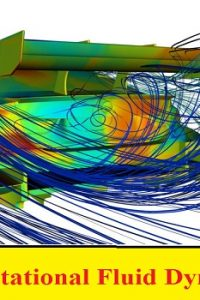اسم المؤلف
Dr. Ahmed Nagib Elmekawy
التاريخ
1 مايو 2020
المشاهدات
التقييم(لا توجد تقييمات)Loading...

محاضرات بعنوان
ME 733 – Computational Fluid Dynamics I – Fall 2018
Dr. Ahmed Nagib Elmekawy
Course Description
• The course is an introductory course to computational fluid dynamics
• Finite difference method will be used to solve different type of Partial
Differential Equations (PDEs) that descript different fluid dynamics
and heat transfer problems.
• Several model equations will be considered for finite difference
discretization, stability and error analysis.
• Solution schemes and boundary conditions treatment will be selected
based on the PDEs classification.
• Scalar form of Navier-Stokes (NS) equations will be solved along with
two-dimensional incompressible NS equations.
• Introduction (What is CFD?, Physical classifications of fluid dynamics
problems, governing equations)
• Classification of PDEs (Characteristics lines and boundary conditions)
• Finite Difference Method (Taylor series, truncation error, consistency,
stability, and convergence)
• Stability analysis
• Parabolic PDEs
• Elliptic PDEs
• Hyperbolic PDEs
• Scalar NS equation
• Introduction to Finite Volume
Introduction to Ansys Fluent
• Intoduction to CFD by using ANSYS
• Introduction to Meshing
• CFD: Setting up Domain and Physics
• CFD: Turbulence Modeling and Transient
References
• Hoffmann, A., Chiang, S., Computational Fluid Dynamics for Engineers, Vol. I, 1st
ed., Engineering Education System, 1993
• Lecture PowerPoint notes
• Hirsch, C., Numerical Computation of Internal and External Flows, 2nd ed.,
Butterworth-Heinemann, 2007
• Anderson, J. D., Computational Fluid Dynamics – The Basics with Applications,
McGraw-Hill, 1995.
• Pletcher, R. H., Tannehill, J. C., Anderson, D., Computational Fluid Mechanics and
Heat Tranfer, 3rd ed., CRC Press, 2011
• Ferziger, J. H., Numerical Methods for Engineering Application, 2nd ed., Wiley,
1998.
• Chung, T.J., Computational Fluid Mechanics, 2nd edition, Cambridge University
Press, 2010.
Classification of Partial Differential Equations
Module 01: Core Skills
Introduction to ANSYS Meshing
Overview
In this lecture we will learn:
• Meshing Fundamentals
• ANSYS Meshing interface
• Geometry concepts
• Meshing methods
• Diagnostics & Usability
• Display Option
• Mesh Statistics & Mesh Metrics
Elliptic PDEs: Finite Difference Formulation
Boundary Conditions
Outline
• Overview.
• Inlet and outlet boundaries.
– Velocity.
– Pressure boundaries and others.
• Wall, symmetry, periodic and axis boundaries.
• Internal cell zones.
– Fluid, porous media, moving cell zones.
– Solid.
• Internal face boundaries.
• Material properties.
• Proper specification.
Turbulence Modelling
Lecture Outline
• Characterization of Turbulent Flows
• Computation of turbulent flows (DNS, LES and RANS approaches)
• Reynolds Averaged Navier-Stokes Equations (RANS)
• Reynolds Stress Tensor and the Closure Problem
• Turbulence Kinetic Energy (k) Equation
• Eddy Viscosity Models (EVM)
• Reynolds Stress Model
• Near-wall Treatments Options and Mesh Requirement
• Inlet Boundary Conditions
• Turbulence Modeling Guidelines
Fluent Solver Settings
Lecture Theme:
Fluent requires inputs (solver settings) which tell it how to calculate the
solution. By introducing the concepts of accuracy, stability and
convergence, the purpose of each setting can be understood. Emphasis
will be placed on convergence, which is critical for the CFD simulation.
Learning Aims:
You will learn:
How to choose the solver and the discretization schemes
How to initialize the solution
How to monitor and judge solution convergence and accuracy
Learning Objectives:
You will be able to choose appropriate solver settings for your CFD
simulation and be able to monitor and judge solution convergence
Introduction
Fluent Best Practices
Lecture Theme:
The accuracy of CFD results can be affected by different types of errors. By understanding the
cause of each different error type, best practices can be developed to minimize them. Meshing
plays a significant role in the effort to minimize errors.
Learning Aims:
You will learn:
• Four different types of errors
• Strategies for minimizing error
• Issues to consider during mesh creation such as quality and cell type
• Best practices for mesh creation
Learning Objectives:
You will understand the causes of error in the solution and how to build the mesh and perform
the simulation in a manner that will minimize errors.
Introduction Error Types Best Practices for Meshing Summary
Introduction
CFD Tutorials
Number Tutorial Name Location Due Date
1 Flow over an Airfoil Link 10/11/2018
2 Flow over an Airfoil at different angle of attack Link 10/11/2018
3 Turbulent Flow past a backward step Tutorial Folder 17/11/2018
4 3D Transonic Flow over a Wing Link 24/11/2018
6 Vertical Axis Wind Turbine (Part 2) – Transient Link 8/12/2018
7 Horizontal Axis Wind Turbine Link 15/12/2018
Meshing Tutorials
Number Tutorial Name Location Due Date
1 Tee Pipe Tutorial Folder 17/11/2018
2 Ventilation System Tutorial Folder 17/11/2018
3 Main Gear Tutorial Folder 24/11/2018
4 Automotive External Body Tutorial Folder 1/12/2018
Tutorial Report Requirements
• Each student Should submit the tutorials at the posted due dates.
• Late Tutorials will not be graded.
• The student should follow all tutorial instructions.
• The Report should include the following
o Problem Description
o Used Boundary Conditions
o Pressure and Velocity Contours
o Comparing with the provided experimental data if provided.
كلمة سر فك الضغط : books-world.net